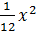### Sample Problem

Given that y =, what is the focus and the directrix?

Focus: ( 0 , )

Directrix: y =

#### Solution

Parabola with vertical axis: (x – h)2 = 4p(y – k), p ≠ 0

Focus is  (h, k+p)directrix is the line  y = k – p.

From the given equation y =,

12y = x2

4(3)y = x2

p = 3

Focus: (0,3)

Directrix is line y = -3